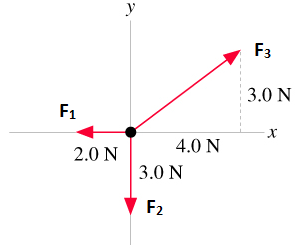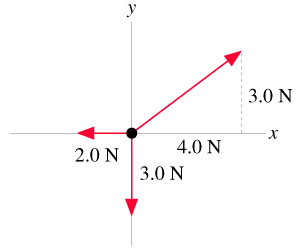# Problem: The forces in (Figure 1) are acting on a 3.0-kg object.What is ax, the x-component of the object's acceleration?Express your answer to two significant figures and include the appropriate units

###### FREE Expert Solution

Newton's second law,

$\overline{){\mathbf{\Sigma }}{\mathbf{F}}{\mathbf{=}}{\mathbf{m}}{\mathbf{a}}}$

The forces acting on the object are,F1 = - 2.0 N i

F2 = - 3.0 N j

F3 = 4.0 N i + 3.0 N j

m = 3.0 kg

80% (244 ratings)###### Problem Details

The forces in (Figure 1) are acting on a 3.0-kg object.What is ax, the x-component of the object's acceleration?

Express your answer to two significant figures and include the appropriate units